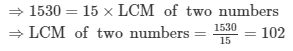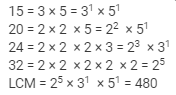Courses

# RD Sharma Solutions (Part - 2) - Whole Number Class 6 Notes | EduRev

## RD Sharma Solutions for Class 6 Mathematics

Created by: Abhishek Kapoor

## Class 6 : RD Sharma Solutions (Part - 2) - Whole Number Class 6 Notes | EduRev

The document RD Sharma Solutions (Part - 2) - Whole Number Class 6 Notes | EduRev is a part of the Class 6 Course RD Sharma Solutions for Class 6 Mathematics.
All you need of Class 6 at this link: Class 6

Ques 9: The product of the predecessor and successor of an odd natural number is always divisible by
(a) 2
(b) 4
(c) 6
(d) 8
Ans: (d) 8
The predecessor of an odd number is an even number.
The successor of an odd number is also an even number.
These two even numbers are two consecutive even numbers, and the product of two
onsecutive even numbers is always divisible by 8.

Ques 10: The product of the predecessor and successor of an even natural number is
(a) divisible by 2
(b) divisible by 3
(c) divisible by 4
(d) an odd number

Ans:
(d) an odd number
Example:

Even natural number = 2

Predecessor of  2 = 2 − 1 = 1

Successor of 2 = 2 + 1 = 3

Their product = 1 × 3 = 3

Thus, the product is an odd number.

Ques 11: The successor of the smallest prime number is
(a) 1
(b) 2
(c) 3
(d) 4

Ans 11:
The smallest prime number is 2
So, Successor of 2 = 2 + 1 = 3
Hence, the correct answer is option (c).

Ques 12: If x and y are co-primes, then their LCM is
(a) 1

(b) x/y
(c) xy
(d) None of these
Ans: A set of numbers which do not have any other common factor other than 1 are called co-prime. The LCM of two co-prime numbers is equal to their product. Hence, the correct answer is option (c).

Ques 13:

The HCF of two co-primes is

(a) the smaller number

(b) the larger number

(c) product of the numbers

(d) 1
Ans:
A set of numbers which do not have any other common factor other than 1 are called co-prime.
The HCF of two co-prime numbers is 1.
Hence, the correct answer is option (d).

Ques 14: The smallest number which is neither prime nor composite is
(a) 0
(b) 1
(c) 2
(d) 3
Ans
: The smallest number which is neither prime nor composite is 1

Hence, the correct answer is option (b).

Ques 15: The product of any natural number and the smallest prime is
(a) an even number
(b) an odd number
(c) a prime number
(d) None of these
Ans:
The smallest prime number is 2.
Thus, when we multiply any natural number we will always get an even number.
Hence, the correct answer is option (a).

Ques 16: Every counting number has an infinite number of

(a) factors

(b) multiples

(c) prime factors

(d) None of these

Ans: Multiples are what we get after multiplying the number by any number.
Thus, every counting number has an infinite number of multiples
Hence, the correct answer is option (b).

Ques 17: The product of two numbers is 1530 and their HCF is 15. The LCM of these numbers is

(a) 102

(b) 120

(c) 84

(d) 112
Ans:
Product of two numbers = HCF of two numbers × LCM of two numbersence, the correct answer is option (a).

Ques 18: The least number divisible by each of the numbers 15, 20, 24 and 32 is
(a) 960
(b) 480
(c) 360
(d) 640
Ans:
LCM of 15, 20, 24 and 32 is given byHence, the correct answer is option (b).

Ques 19: The greatest number which divides 134 and 167 leaving 2 as remainder in each case is
(a) 14
(b) 19
(c) 33
(d) 17
Ans:First we subtract the required remainder from 134 and 167.
Thus, we will get 132 and 165.
132 = 2 × 2 × 3 × 11 = 22 × 3 × 11
165 = 3 × 5 × 11 = 31 × 5 × 11
HCF = 3 × 11 = 33
Thus, the greatest number which divides 134 and 167 leaving 2 as remainder in each case is 33
Hence, the correct answer is option (c).

Ques 20: Which of the following numbers is a prime number?
(a) 91
(b) 81
(c) 87
(d) 97
Ans:
Since, factors of
91 = 1 × 7 × 13
81 = 1 × 3 × 3 × 3 × 3
87 = 1 × 3 × 29
97 = 1 × 97
Thus, 81, 87 and 91 all are not prime numbers.
Hence, the correct answer is option (d).

Ques 21: If two numbers are equal, then
(a) their LCM is equal to their HCF
(b) their LCM is less than their HCF
(c) their LCM is equal to two times their HCF
(d) None of these
Ans:
If two numbers are equal, then their LCM is equal to their HCF
Hence, the correct answer is option (a).

Ques 22: a and b are two co-primes. Which of the following is/are true?
(a) LCM (a, b) = a × b
(b) HCF (a, b) = 1
(c) Both (a) and (b)
(d) Neither (a) nor (b)
Ans 22: A set of numbers which do not have any other common factor other than 1 are called co-prime.
The LCM of two co-prime numbers is equal to their product.
The HCF of two co-prime numbers is 1.
Hence, the correct answer is option (c).

103 docs

,

,

,

,

,

,

,

,

,

,

,

,

,

,

,

,

,

,

,

,

,

;